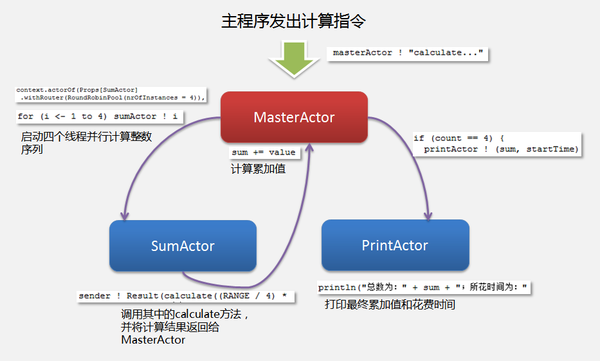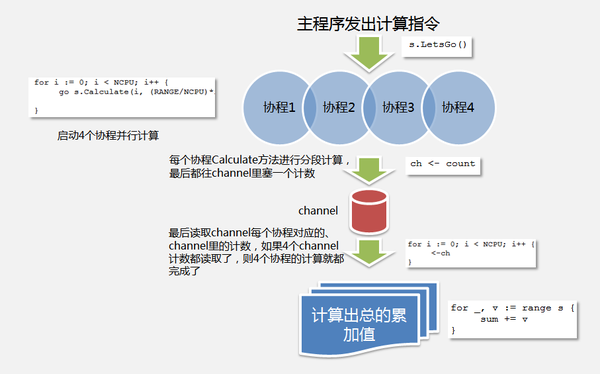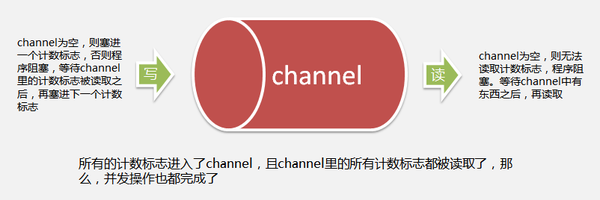# Scala与Golang的并发实现对比

2gua · · 18503 次点击 · · 开始浏览

Scala语言并发设计采用Actor模型，借鉴了Erlang的Actor实现，并且在Scala 2.10之后，Scala采用的是Akka Actor模型库。Actor模型主要特征如下：
1. “一切皆是参与者”，且各个actor间是独立的；
2. 发送者与已发送消息间解耦，这是Actor模型显著特点，据此实现异步通信；
3. actor是封装状态和行为的对象，通过消息交换进行相互通信，交换的消息存放在接收方的邮箱中；
4. actor可以有父子关系，父actor可以监管子actor，子actor唯一的监管者就是父actor；
5. 一个actor就是一个容器，它包含了状态、行为、一个邮箱（邮箱用来接受消息）、子actor和一个监管策略；
Go语言也能够实现传统的共享内存的通信方式，但Go更提倡“以通信来共享内存，而非以共享内存来通信”。Go的并发通信方式借鉴CSP(Communicating Sequential Process)模型，其主要特征如下：
1. goroutine（协程，Go的轻量级线程）是Go的轻量级线程管理机制，用“go”启动一个goroutine， 如果当前线程阻塞则分配一个空闲线程，如果没有空闲线程，则新建一个线程；
2. 通过管道（channel）来存放消息，channel在goroutine之间传递消息；比如通过读取channel里的消息（通俗点说好比一个个“值”），你能够明白某个goroutine里的任务完成以否；
3. Go给channel做了增强，可带缓存。
Scala与Go在并发通信模型实现上的主要差异如下：
1. actor是异步的，因为发送者与已发送消息间实现了解耦；而channel则是某种意义上的同步，比如channel的读写是有关系的，期间会依赖对方来决定是否阻塞自己；
2. actor是一个容器，使用actorOf来创建Actor实例时，也就意味着需指定具体Actor实例，即指定哪个actor在执行任务，该actor必然要有“身份”标识，否则怎么指定呢？！而channel通常是匿名的， 任务放进channel之后你不用关心是哪个channel在执行任务；

``````// Akka并发计算实例

import akka.actor.Actor
import akka.actor.Props
import akka.actor.ActorSystem
import akka.routing.RoundRobinPool

// 定义一个case类
sealed trait SumTrait
case class Result(value: Int) extends SumTrait

// 计算用的Actor
class SumActor extends Actor {
val RANGE = 10000

def calculate(start: Int, end: Int, flag : String): Int = {
var cal = 0

for (i <- (start to end)) {
for (j <- 1 to 3000000) {}
cal += i
}

println("flag : " + flag + ".")
return cal
}

case value: Int =>
sender ! Result(calculate((RANGE / 4) * (value - 1) + 1, (RANGE / 4) * value, value.toString))
case _ => println("未知 in SumActor...")
}
}

// 打印结果用的Actor
class PrintActor extends Actor {
case (sum: Int, startTime: Long) =>
println("总数为：" + sum + "；所花时间为："
+ (System.nanoTime() - startTime)/1000000000.0 + "秒。")
case _ => println("未知 in PrintActor...")
}
}

// 主actor，发送计算指令给SumActor，发送打印指令给PrintActor
class MasterActor extends Actor {
var sum = 0
var count = 0
var startTime: Long = 0

// 声明Actor实例，nrOfInstances是pool里所启routee（SumActor）的数量，
// 这里用4个SumActor来同时计算，很Powerful。
val sumActor   = context.actorOf(Props[SumActor]
.withRouter(RoundRobinPool(nrOfInstances = 4)), name = "sumActor")
val printActor = context.actorOf(Props[PrintActor], name = "printActor")

case "calculate..." =>
startTime = System.nanoTime()
for (i <- 1 to 4) sumActor ! i
case Result(value) =>
sum += value
count += 1
if (count == 4) {
printActor ! (sum, startTime)
context.stop(self)
}
case _ => println("未知 in MasterActor...")
}
}

object Sum {
def main(args: Array[String]): Unit = {
var sum = 0

val system = ActorSystem("MasterActorSystem")
val masterActor = system.actorOf(Props[MasterActor], name = "masterActor")
masterActor ! "calculate..."

system.shutdown()
}
}
``````在这里，我们一共定义了 三个Actor实例（actor），MasterActor、SumActor和PrintActor，其中，前者是后两者的父亲actor，如前文Scala的Actor模型特征里提到的：“actor可以有父子关系，父actor可以监管子actor，子actor唯一的监管者就是父actor”。

``````val sumActor   = context.actorOf(Props[SumActor]
.withRouter(RoundRobinPool(nrOfInstances = 4)), name = "sumActor")
``````

``````for (i <- 1 to 4) sumActor ! i
``````

Scala Akka的并发实现，给我的感觉是设计才是关键，将各个actor的功能及关联关系表述清楚，剩余的代码实现就非常容易，这正是Scala、Akka的魅力体现，在底层帮我们做了大量工作！

``````...
val RANGE = 10000
var cal = 0
val startTime = System.nanoTime()

for (i <- (1 to RANGE)) {
for (j <- 1 to 3000000) {}
cal += i
}

val endTime = System.nanoTime()
...
``````

``````// Go并发计算实例

package main

import (
"fmt"
"runtime"
"strconv"
"time"
)

type Sum []int

func (s Sum) Calculate(count, start, end int, flag string, ch chan int) {
cal := 0

for i := start; i <= end; i++ {
for j := 1; j <= 3000000; j++ {
}
cal += i
}

s[count] = cal
fmt.Println("flag :", flag, ".")
ch <- count
}

func (s Sum) LetsGo() {
// runtime.NumCPU()可以获取CPU核数，我的环境为4核，所以这里就简单起见直接设为4了
const NCPU = 4
const RANGE = 10000
var ch = make(chan int)

runtime.GOMAXPROCS(NCPU)
for i := 0; i < NCPU; i++ {
go s.Calculate(i, (RANGE/NCPU)*i+1, (RANGE/NCPU)*(i+1), strconv.Itoa(i+1), ch)
}

for i := 0; i < NCPU; i++ {
<-ch
}
}

func main() {
var s Sum = make([]int, 4, 4)
var sum int = 0
var startTime = time.Now()

s.LetsGo()

for _, v := range s {
sum += v
}

fmt.Println("总数为：", sum, "；所花时间为：",
(time.Now().Sub(startTime)), "秒。")
}
``````
Go语言的实现与之前的Scala实现风格完全不一样，其通过“go”关键字实现的goroutine协程工作方式，结合channel，实现并发功能。goroutine和channel是Go语言非常强大的两个招式，简约而不简单。在这里，我们的并发实现模型如下图所示：由上可知，Go语言的并发魔力来源于goroutine和channel。我们定义了一个Sum类型（插一句：Go语言的类型系统设计得也非常特别，这是别的主题了,:)），它有两个方法：LetsGo()和Calculate，LetsGo()首先创建一个计数用的channel，随后发起4个并发计算的协程。每个计算协程调用Calculate()进行分段计算（并会传入channel），Calculate()方法的最后，在分段计算完成时，都会往channel里塞一个计数标志：
``````ch <- count
``````

``````for i := 0; i < NCPU; i++ {
<-ch
}
``````这里为了演示方便，且本例中的协程和业务逻辑也不至于会造成协程僵死或locked，因此未考虑协程永久等待的处理，如果要处理超时，可以这么考虑：

``````for {
select {
case <-ch: ...
case <-time.After(3 * time.Second): ...
}
}
``````

select机制也是Go语言并发处理中的强大武器，由于与本主题关系不大，故不表。但可以看出，Go语言有Unix和C的深深烙印，select、channel概念就是很好的例证。

``````for _, v := range s {
sum += v
}
``````

Go中的channel是可以带缓存的，在缓存未被填满之前，都可以写入。本例中未使用带缓存的channel，虽然这样做在理论上可以节省写入channel时的等待时间，但在这里可以忽略，大型应用中就要慎重对待了。

``````...
cal := 0

for i := start; i <= end; i++ {
for j := 1; j <= 3000000; j++ {
}
cal += i
}
...
``````

``````scalac -cp lib\akka-actor_2.11-2.3.4.jar Sum.scala
scala Sum

go build Sum.go
Sum
``````

Scala：7.189461763秒（单线程模式），3.895642655秒（并发模式）
Golang 12.987232秒（单线程模式），7.1636263秒（并发模式）

1. Akka的actor是解耦的、相对独立的，定义好各个actor间如何沟通，剩下的东西就尽管交给它们处理好了，它们自会按既定方式各司其职，而且每个actor“麻雀虽小五脏俱全”，这也是其解耦性做得好的必然基础。Go语言则独辟蹊径，通过“go”魔法和Unix风格的channel，以更轻量级的协程方式来处理并发，虽说是更轻量，但你仍得花点心思关注下channel的状态，别一不小心阻塞了，特别是channel多了、复杂了，并且其中包含了业务处理所需数据、而不仅仅只是计数标志的情况下；
2. Akka的Actor实现是库的形式，其也能应用于Java语言。Go语言内嵌了协程的并发实现；
3. Akka基于JVM，实现模式是面向对象，天然讲究抽象与封装，虽然可以穿插混合应用函数式风格。而Go语言显然体现了命令式语言的风格，在需要考虑封装性的时候，需要开发者多着墨。0 回复

• 请尽量让自己的回复能够对别人有帮助
• 支持 Markdown 格式, **粗体**、~~删除线~~、``单行代码``
• 支持 @ 本站用户；支持表情（输入 : 提示），见 Emoji cheat sheet
• 图片支持拖拽、截图粘贴等方式上传

Scala语言并发设计采用Actor模型，借鉴了Erlang的Actor实现，并且在Scala 2.10之后，Scala采用的是Akka Actor模型库。Actor模型主要特征如下：
1. “一切皆是参与者”，且各个actor间是独立的；
2. 发送者与已发送消息间解耦，这是Actor模型显著特点，据此实现异步通信；
3. actor是封装状态和行为的对象，通过消息交换进行相互通信，交换的消息存放在接收方的邮箱中；
4. actor可以有父子关系，父actor可以监管子actor，子actor唯一的监管者就是父actor；
5. 一个actor就是一个容器，它包含了状态、行为、一个邮箱（邮箱用来接受消息）、子actor和一个监管策略；
Go语言也能够实现传统的共享内存的通信方式，但Go更提倡“以通信来共享内存，而非以共享内存来通信”。Go的并发通信方式借鉴CSP(Communicating Sequential Process)模型，其主要特征如下：
1. goroutine（协程，Go的轻量级线程）是Go的轻量级线程管理机制，用“go”启动一个goroutine， 如果当前线程阻塞则分配一个空闲线程，如果没有空闲线程，则新建一个线程；
2. 通过管道（channel）来存放消息，channel在goroutine之间传递消息；比如通过读取channel里的消息（通俗点说好比一个个“值”），你能够明白某个goroutine里的任务完成以否；
3. Go给channel做了增强，可带缓存。
Scala与Go在并发通信模型实现上的主要差异如下：
1. actor是异步的，因为发送者与已发送消息间实现了解耦；而channel则是某种意义上的同步，比如channel的读写是有关系的，期间会依赖对方来决定是否阻塞自己；
2. actor是一个容器，使用actorOf来创建Actor实例时，也就意味着需指定具体Actor实例，即指定哪个actor在执行任务，该actor必然要有“身份”标识，否则怎么指定呢？！而channel通常是匿名的， 任务放进channel之后你不用关心是哪个channel在执行任务；

``````// Akka并发计算实例

import akka.actor.Actor
import akka.actor.Props
import akka.actor.ActorSystem
import akka.routing.RoundRobinPool

// 定义一个case类
sealed trait SumTrait
case class Result(value: Int) extends SumTrait

// 计算用的Actor
class SumActor extends Actor {
val RANGE = 10000

def calculate(start: Int, end: Int, flag : String): Int = {
var cal = 0

for (i <- (start to end)) {
for (j <- 1 to 3000000) {}
cal += i
}

println("flag : " + flag + ".")
return cal
}

case value: Int =>
sender ! Result(calculate((RANGE / 4) * (value - 1) + 1, (RANGE / 4) * value, value.toString))
case _ => println("未知 in SumActor...")
}
}

// 打印结果用的Actor
class PrintActor extends Actor {
case (sum: Int, startTime: Long) =>
println("总数为：" + sum + "；所花时间为："
+ (System.nanoTime() - startTime)/1000000000.0 + "秒。")
case _ => println("未知 in PrintActor...")
}
}

// 主actor，发送计算指令给SumActor，发送打印指令给PrintActor
class MasterActor extends Actor {
var sum = 0
var count = 0
var startTime: Long = 0

// 声明Actor实例，nrOfInstances是pool里所启routee（SumActor）的数量，
// 这里用4个SumActor来同时计算，很Powerful。
val sumActor   = context.actorOf(Props[SumActor]
.withRouter(RoundRobinPool(nrOfInstances = 4)), name = "sumActor")
val printActor = context.actorOf(Props[PrintActor], name = "printActor")

case "calculate..." =>
startTime = System.nanoTime()
for (i <- 1 to 4) sumActor ! i
case Result(value) =>
sum += value
count += 1
if (count == 4) {
printActor ! (sum, startTime)
context.stop(self)
}
case _ => println("未知 in MasterActor...")
}
}

object Sum {
def main(args: Array[String]): Unit = {
var sum = 0

val system = ActorSystem("MasterActorSystem")
val masterActor = system.actorOf(Props[MasterActor], name = "masterActor")
masterActor ! "calculate..."

system.shutdown()
}
}
``````在这里，我们一共定义了 三个Actor实例（actor），MasterActor、SumActor和PrintActor，其中，前者是后两者的父亲actor，如前文Scala的Actor模型特征里提到的：“actor可以有父子关系，父actor可以监管子actor，子actor唯一的监管者就是父actor”。

``````val sumActor   = context.actorOf(Props[SumActor]
.withRouter(RoundRobinPool(nrOfInstances = 4)), name = "sumActor")
``````

``````for (i <- 1 to 4) sumActor ! i
``````

Scala Akka的并发实现，给我的感觉是设计才是关键，将各个actor的功能及关联关系表述清楚，剩余的代码实现就非常容易，这正是Scala、Akka的魅力体现，在底层帮我们做了大量工作！

``````...
val RANGE = 10000
var cal = 0
val startTime = System.nanoTime()

for (i <- (1 to RANGE)) {
for (j <- 1 to 3000000) {}
cal += i
}

val endTime = System.nanoTime()
...
``````

``````// Go并发计算实例

package main

import (
"fmt"
"runtime"
"strconv"
"time"
)

type Sum []int

func (s Sum) Calculate(count, start, end int, flag string, ch chan int) {
cal := 0

for i := start; i <= end; i++ {
for j := 1; j <= 3000000; j++ {
}
cal += i
}

s[count] = cal
fmt.Println("flag :", flag, ".")
ch <- count
}

func (s Sum) LetsGo() {
// runtime.NumCPU()可以获取CPU核数，我的环境为4核，所以这里就简单起见直接设为4了
const NCPU = 4
const RANGE = 10000
var ch = make(chan int)

runtime.GOMAXPROCS(NCPU)
for i := 0; i < NCPU; i++ {
go s.Calculate(i, (RANGE/NCPU)*i+1, (RANGE/NCPU)*(i+1), strconv.Itoa(i+1), ch)
}

for i := 0; i < NCPU; i++ {
<-ch
}
}

func main() {
var s Sum = make([]int, 4, 4)
var sum int = 0
var startTime = time.Now()

s.LetsGo()

for _, v := range s {
sum += v
}

fmt.Println("总数为：", sum, "；所花时间为：",
(time.Now().Sub(startTime)), "秒。")
}
``````
Go语言的实现与之前的Scala实现风格完全不一样，其通过“go”关键字实现的goroutine协程工作方式，结合channel，实现并发功能。goroutine和channel是Go语言非常强大的两个招式，简约而不简单。在这里，我们的并发实现模型如下图所示：由上可知，Go语言的并发魔力来源于goroutine和channel。我们定义了一个Sum类型（插一句：Go语言的类型系统设计得也非常特别，这是别的主题了,:)），它有两个方法：LetsGo()和Calculate，LetsGo()首先创建一个计数用的channel，随后发起4个并发计算的协程。每个计算协程调用Calculate()进行分段计算（并会传入channel），Calculate()方法的最后，在分段计算完成时，都会往channel里塞一个计数标志：
``````ch <- count
``````

``````for i := 0; i < NCPU; i++ {
<-ch
}
``````这里为了演示方便，且本例中的协程和业务逻辑也不至于会造成协程僵死或locked，因此未考虑协程永久等待的处理，如果要处理超时，可以这么考虑：

``````for {
select {
case <-ch: ...
case <-time.After(3 * time.Second): ...
}
}
``````

select机制也是Go语言并发处理中的强大武器，由于与本主题关系不大，故不表。但可以看出，Go语言有Unix和C的深深烙印，select、channel概念就是很好的例证。

``````for _, v := range s {
sum += v
}
``````

Go中的channel是可以带缓存的，在缓存未被填满之前，都可以写入。本例中未使用带缓存的channel，虽然这样做在理论上可以节省写入channel时的等待时间，但在这里可以忽略，大型应用中就要慎重对待了。

``````...
cal := 0

for i := start; i <= end; i++ {
for j := 1; j <= 3000000; j++ {
}
cal += i
}
...
``````

``````scalac -cp lib\akka-actor_2.11-2.3.4.jar Sum.scala
scala Sum

go build Sum.go
Sum
``````

Scala：7.189461763秒（单线程模式），3.895642655秒（并发模式）
Golang 12.987232秒（单线程模式），7.1636263秒（并发模式）

1. Akka的actor是解耦的、相对独立的，定义好各个actor间如何沟通，剩下的东西就尽管交给它们处理好了，它们自会按既定方式各司其职，而且每个actor“麻雀虽小五脏俱全”，这也是其解耦性做得好的必然基础。Go语言则独辟蹊径，通过“go”魔法和Unix风格的channel，以更轻量级的协程方式来处理并发，虽说是更轻量，但你仍得花点心思关注下channel的状态，别一不小心阻塞了，特别是channel多了、复杂了，并且其中包含了业务处理所需数据、而不仅仅只是计数标志的情况下；
2. Akka的Actor实现是库的形式，其也能应用于Java语言。Go语言内嵌了协程的并发实现；
3. Akka基于JVM，实现模式是面向对象，天然讲究抽象与封装，虽然可以穿插混合应用函数式风格。而Go语言显然体现了命令式语言的风格，在需要考虑封装性的时候，需要开发者多着墨。